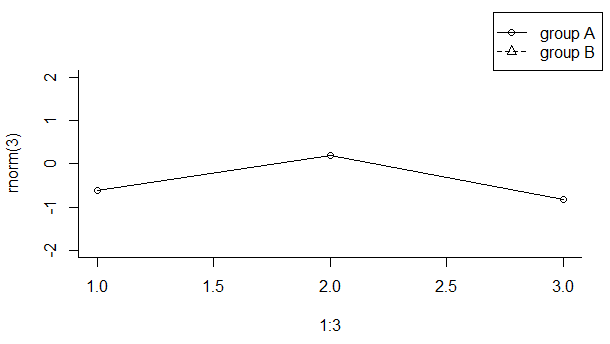2 views

As the title says: How can I plot a legend outside the plotting area when using base graphics?

I thought about fiddling around with the layout and produce an empty plot to only contain the legend, but I would be interested in a way using just the base graph facilities and e.g., par(mar = ) to get some space on the right of the plot for the legend.

Here an example:

plot(1:3, rnorm(3), pch = 1, lty = 1, type = "o", ylim=c(-2,2))

lines(1:3, rnorm(3), pch = 2, lty = 2, type="o")

legend(1,-1,c("group A", "group B"), pch = c(1,2), lty = c(1,2))

produces:But as said, I would like the legend to be outside the plotting area (e.g., to the right of the graph/plot.

by

To plot a legend outside the plotting area in base graphics, you can set the xpd argument of the par function.

According to R  Documentation:

xpd

A logical value or NA. If FALSE, all plotting is clipped to the plot region, if TRUE, all plotting is clipped to the figure region, and if NA, all plotting is clipped to the device region.

set.seed(1)

plot(1:3, rnorm(3), pch = 1, lty = 1, type = "o", ylim=c(-2,2), bty='L')

par(xpd=TRUE)

legend(2.7,3.5,c("group A", "group B"), pch = c(1,2), lty = c(1,2))

Output: